# 2. Measuring Video Similarity

## 2. Measuring Video Similarity

This section defines the video similarity models used in this chapter, and describes how they can be efficiently estimated by our proposed algorithms. We assume that individual frames in a video are represented by high-dimensional feature vectors from a metric space (F,d( )). In order to be robust against editing changes in temporal domain, we define a video X as a finite set of feature vectors and ignore any temporal ordering. For the remainder of this chapter, we make no distinction between a video frame and its corresponding feature vector. The metric d(x, y) measures the visual dissimilarity between frames x and y. We assume that frames x and y are visually similar to each other if and only if d(x, y)≤ε for an ε > 0 independent of x and y . We call ε the Frame Similarity Threshold.

In Section 2.1, we define our target measure, called the Ideal Video Similarity (IVS), used in this chapter to gauge the visual similarity between two video sequences. As we explain in the section, this similarity measure is complex to compute exactly, and requires a significant number of frames to represent each video. To reduce the computational complexity and the representation size, we propose the ViSig method in Section 2.3 to estimate the IVS. We provide an analytical bound on the number of frames required to represent each video in our proposed algorithm. From Sections 2.4 through 2.7, we analyze the scenarios where IVS cannot be reliably estimated by our proposed algorithm, and propose a number of heuristics to rectify the problems.

### 2.1 Ideal Video Similarity (IVS)

As mentioned in Section 1, we are interested in defining a video similarity measure that is based on the percentage of visually similar frames between two sequences. A naive way to compute such a measure is to first find the total number of frames from each video sequence that have at least one visually similar frame in the other sequence, and then compute the ratio of this number to the overall total number of frames. We call this measure the Na ve Video Similarity (NVS):

##### Definition 1. Na ve Video Similarity

Let X and Y be two video sequences. The number of frames in video X that have at least one visually similar frame in Y is represented by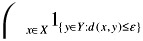, where 1A is the indicator function with 1A = 1 if A is not empty, and zero otherwise. The Na ve Video Similarity between X and Y, nvs(X,Y;ε), can thus be defined as follows:

 (28.1)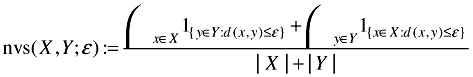If every frame in video X has a similar match in Y and vice versa, nvs(X,Y;ε) = 1. If X and Y share no similar frames at all, nvs(X,Y;ε) = 0. Unfortunately, NVS does not always reflect our intuition of video similarity. Most real-life video sequences can be temporally separated into video shots, within which the frames are visually similar. Among all possible versions of the same video, the number of frames in the same shot can be quite different. For instance, different coding schemes modify the frame rates for different playback capabilities, and video summarization algorithms use a single key-frame to represent an entire shot. As NVS is based solely on frame counts, its value is highly sensitive to these kinds of manipulations. To illustrate this with a pathological example, consider the following: given a video X, create a video Y by repeating one single frame in X for a great many times. If |Y| >> |X|, nvs(X, Y;ε) 1 even though X and Y share one common frame. It is possible to rectify the problem by using shots as the fundamental unit for similarity measurement. Since we model a video as a set and ignore all temporal ordering, we instead group all visually similar frames in a video together into non-intersecting units called clusters. A cluster should ideally contain only similar frames, and no other frames similar to the frames in a cluster should be found in the rest of the video. Mathematically, we can express these two properties as follows: for all pairs of frames xi and xj in X, d(xi,xj)ε if and only if xi and xj belong to the same cluster. Unfortunately, such a clustering structure may not exist for an arbitrary video X. Specifically, if d(xi,xj) ε and d(xj,xk) ε, there is no guarantee that d(xi,xk) ε. If d(xi,xk) > ε, there is no consistent way to group all the three frames into clusters.

In order to arrive at a general framework for video similarity, we adopt a relatively relaxed clustering structure by only requiring the forward condition, i.e., d(xi,xj) ε implies that xi and xj are in the same cluster. A cluster is simply one of the connected components  of a graph in which each node represents a frame in the video, and every pair of frames within ε from each other are connected by an edge. We denote the collection of all clusters in video X as [X]ε. It is possible for such a definition to produce chain-like clusters where one end of a cluster is very far from the other end. Nonetheless, given an appropriate feature vector and a reasonably small ε, we have empirically found most clusters in real video sequences to be compact, i.e., all frames in a cluster are similar to each other. We call a cluster ε -compact if all its frames are within ε from each other. The clustering structure of a video can be computed by a simple hierarchical clustering algorithm called the single-link algorithm .

In order to define a similarity measure based on the visually similar portion shared between two video sequences X and Y, we consider the clustered union [XY]ε. If a cluster in [XY]ε contains frames from both sequences, these frames are likely to be visually similar to each other. Thus, we call such a cluster Similar Cluster and consider it as part of the visually similar portion. The ratio between the number of Similar Clusters and the total number of clusters in [XY]ε forms a reasonable similarity measure between X and Y. We call this measure the Ideal Video Similarity (IVS):

##### Definition 2. Ideal Video Similarity

Let X and Y be two video sequences. The IVS between X and Y, or ivs(X,Y;ε), is defined to be the fraction of clusters in [XY]ε that contain frames from sequences, i.e., C[XY]ε with lCX 1CY = 1. Specifically ivs(X,Y;ε) can be expressed by the following equation:

 (28.2)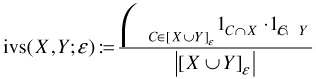The main theme of this chapter is to develop efficient algorithms to estimate the IVS between a pair of video sequences. A simple pictorial example to demonstrate the use of IVS is shown in Figure 28.1 (a). The feature space is represented as a 2D square. Dots and crosses signify frames from two different video sequences, and frames closer than ε are connected by dotted lines. There are altogether three clusters in the clustered union, and only one cluster has frames from both sequences. The IVS is thus 1/3.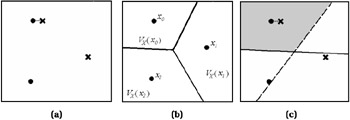Figure 28.1: (a) Two video sequences with IVS equal to 1/3. (b) The Voronoi Diagram of a 3-frame video X. (c) The shaded area, normalized by the area of the entire space, is equal to the VVS between the two sequences shown.

It is complex to precisely compute the IVS. The clustering used in IVS depends on the distances between frames from the two sequences. This implies that for two l -frame video sequences, one needs to first compute the distance between l2 pairs of frames before running the clustering algorithm and computing the IVS. In addition, the computation requires the entire video to be stored. The complex computation and large storage requirements are clearly undesirable for large database applications. As the exact similarity value is often not required in many applications, sampling techniques can be used to estimate IVS. Consider the following simple sampling scheme: let each video sequence in the database be represented by m randomly selected frames. We estimate the IVS between two sequences by counting the number of similar pairs of frames Wm between their respective sets of sampled frames. As long as the desired level of precision is satisfied, m should be chosen as small as possible to achieve low complexity. Nonetheless, even in the case when the IVS is as large as one, we show in the following proposition that we need a large m to find even one pair of similar frames among the sampled frames.

##### Proposition 1

Let X and Y be two l -frame video sequences. Assume for every frame x in X , Y has exactly one frame y similar to it, i.e., d(x, y) ε. We also assume the same for every frame in Y. Clearly, ivs(X, Y;ε) = 1. The expectation of the number of similar frame pairs Wm found between m randomly selected frames from X and from Y is given below:

 (28.3)Despite the fact that the IVS between the video sequences is one, Equation (28.3) shows that we need, on average,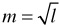sample frames from each video to find just one similar pair. Furthermore, comparing two sets offrames requires l high-dimensional metric computations. A better random sampling scheme should use a fixed-size record to represent each video, and require far fewer frames to identify highly similar video sequences. Our proposed ViSig method is precisely such a scheme and is the topic of the following section.

### 2.2 Voronoi Video Similarity

As described in the previous section, the simple sampling scheme requires a large number of frames sampled from each video to estimate IVS. The problem lies in the fact that since we sample frames from two video sequences independently, the probability that we simultaneously sample a pair of similar frames from them is rather small. Rather than independent sampling, the ViSig method introduces dependence by selecting frames in each video that are similar to a set of predefined random feature vectors common to all video sequences. As a result, the ViSig method takes far fewer sampled frames to find a pair of similar frames from two video sequences. The number of pairs of similar frames found by the ViSig method depends strongly on the IVS, but does not have a one-to-one relationship with it. We call the form of similarity estimated by the ViSig method the Voronoi Video Similarity (VVS). In this section, we explain VVS and, in Section 2.3, we discuss how it is estimated by the ViSig method. The discrepancies between VVS and IVS, and how they can be rectified by modifying the ViSig method are addressed in Sections 2.4 through 2.7.

The term "Voronoi" in VVS is borrowed from a geometrical concept called the Voronoi Diagram. Given a l -frame video X = {xt :t = 1,...,l}, the Voronoi Diagram V(X) of X is a partition of the feature space F into l Voronoi Cells VX(xt). By definition, the Voronoi Cell VX(xt) contains all the vectors in F closer to xt X than to any other frames in X, i.e., VX(xt) := {s F : gX(s) = xt}, where gX(s) denotes the frame in X closest  to s. A simple Voronoi Diagram of a 3-frame video is shown in Figure 28.1(b). We can extend the idea of the Voronoi Diagram to video clusters by merging Voronoi Cells of all the frames belonging to the same cluster. In other words, for C [X]ε, VX(C):=xCVX(x). Given two video sequences X and Y and their corresponding Voronoi Diagrams, we define the Similar Voronoi Region R(X,Y;ε) as the union of all the intersections between the Voronoi Cells of those x X and y Y where d(x, y) ε:

 (28.4)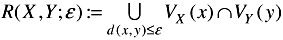It is easy to see that if x and y are close to each other, their corresponding Voronoi Cells are very likely to intersect in the neighborhood of x and y. The larger number of frames from X and from Y that are close to each other, the larger the resulting R(X,Y;ε) becomes. A simple pictorial example of two video sequences with their Voronoi Diagrams is shown in Figure 28.1(c): dots and crosses represent the frames of the two sequences; the solid and broken lines are the boundary between the two Voronoi Cells of the two sequences represented by dots and crosses respectively. The shaded region shows the Similar Voronoi Region between these two sequences. Similar Voronoi Region is the target region whose volume defines VVS. Before providing a definition of VVS, we need to first clarify what we mean by the volume of a region in the feature space.

We define volume function Vol: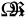to be the Lebesgue measure over the set, Ω, of all the measurable subsets in feature space F . For example, if F is the real line and the subset is an interval, the volume function of the subset is just the length of the interval. We assume all the Voronoi Cells considered in our examples to be measurable. We further assume that F is compact in the sense that Vol(F) is finite. As we are going to normalize all volume measurements by Vol(F), we simply assume that Vol(F) = 1. To compute the volume of the Similar Voronoi Region R(X,Y;ε) between two video sequences X and Y, we first notice that individual terms inside the union in Equation (28.4) are disjoint from each other. By the basic properties of Lebesgue measure, we have

 (28.5)Thus, we define the Voronoi Video Similarity (VVS), vvs(X,Y;ε), between two video sequences X and Y as,

 (28.6)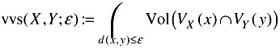The VVS of the two sequences shown in Figure 28.1(c) is the area of the shaded region, which is about 1/3 of the area of the entire feature space. Notice that for this example, the IVS is also 1/3. VVS and IVS are close to each other because the Voronoi Cell for each cluster in the cluster union has roughly the same volume (area).

In general, when the clusters are not uniformly distributed over the feature space, there can be a large variation among the volumes of the corresponding Voronoi Cells. Consequently, VVS can be quite different from IVS. In the following section, we ignore the differences between VVS and IVS and introduce the Basic ViSig method to estimate either similarity measure.

### 2.3 Video Signature Method

It is straightforward to estimate vvs(X, Y;ε) by random sampling: First, generate a set S of m independent uniformly distributed random vectors s1,s2,,sm, which we call Seed Vectors (SV). By uniform distribution, we mean for every measurable subset A in F, the probability of generating a vector from A is Vol(A). Second, for each random vector s S, determine if s is inside R(X,Y;ε). By definition, s is inside R(X,Y;ε) if and only if s belongs to some Voronoi Cells VX(x) and VY(y) with d(x, y) ε. Since s must be inside the Voronoi Cell of the frame closest to s in the entire video sequence, i.e., gX(s) in X and gY(s) in Y, an equivalent condition for s R(X,Y;ε) is d(gX(s), gY(s)) ε. Since we only require gX(s) and gY(s) to determine if each SV s belongs to R(X,Y;ε), we can summarize video X by the m-tuple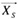:= (gX(s1), gX(s2),, gX(sm)) and Y by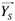:= (gY(s1), gY(s2),..., gY(sm)). We callandthe Video Signature (ViSig) with respect to S of video sequences X and Y respectively. In the final step, we compute the percentage of ViSig frame pairs gX(s) and gY(s) with distances less than or equal to ε to obtain:

 (28.7)We call vssb(,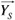;ε,m) the Basic ViSig Similarity (VSSb) between ViSig's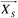and. As every SV s S in the above algorithm is chosen to be uniformly distributed, the probability of s being inside R(X,Y;ε) is Vol(R(X,Y;ε)) = vvs(X,Y;ε). Thus, vssb (,;ε,m) forms an unbiased estimator of the VVS between X and Y. We refer to this approach of generating ViSig and computing VSSb the Basic ViSig method. In order to apply the Basic ViSig method to a large number of video sequences, we must use the same SV set S to generate all the ViSig's in order to compute VSSb between an arbitrary pair of video sequences.

The number of SV's in S, m, is an important parameter. On one hand, m represents the number of samples used to estimate the underlying VVS and thus, the larger m is, the more accurate the estimation becomes. On the other hand, the complexity of the Basic ViSig method directly depends on m. If a video has l frames, it takes l metric computations to generate a single ViSig frame. The number of metric computations required to compute the entire ViSig is thus m l. Also, computing the VSSb between two ViSig's requires m metric computations. It is, therefore, important to determine an appropriate value of m that can satisfy both the desired fidelity of estimation and the computational resource of a particular application. The following proposition provides an analytical bound on m in terms of the maximum error in estimating the VVS between any pair of video sequences in a database:

##### Proposition 2

Assume we are given a database with n video sequences and a set S of m random SV's. Define the error probability Perr(m) to be the probability that any pair of video sequences in has their m -frame VSSb different from the true VVS value by more than a given γ (0,1], i.e.,

 (28.8)A sufficient condition to achieve Perr(m) δ for a given δ (0,1] is as follows:

 (28.9)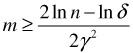It should be noted that the bound (28.9) in Proposition 2 only provides a sufficient condition and does not necessarily represent the tightest bound possible. Nonetheless, we can use such a bound to understand the dependencies of m on various factors. First, unlike the random sampling described in Section 5, m does not depend on the length of individual video sequences. This implies that it takes far fewer frames for the ViSig method to estimate the similarity between long video sequences than random frame sampling. Second, we notice that the bound on m increases with the natural logarithm of n, the size of the database. The ViSig size depends on n because it has to be large enough to simultaneously minimize the error of all possible pairs of comparisons, which is a function of n. Fortunately, the slow-growing logarithm makes the ViSig size rather insensitive to the database size, making it suitable for very large databases. The contribution of the term ln δ is also quite insignificant. Comparatively, m is most sensitive to the choice of γ. A small γ means an accurate approximation of the similarity, but usually at the expense of a large number of sample frames m to represent each video. The choice of γ should depend on the particular application at hand.

### 2.4 Seed Vector Generation

We have shown in the previous section that the VVS between two video sequences can be efficiently estimated by the Basic ViSig method. Unfortunately, the estimated VVS does not necessarily reflect the target measure of IVS as defined in Equation (28.2). For example, consider the two pairs of sequences in Figure 28.2(a) and Figure 28.2(b). As in Figure 28.1(c), dots and crosses are frames from the two sequences, whose Voronoi Diagrams are indicated by solid and broken lines respectively. The IVS's in both cases are 1/3. Nonetheless, the VVS in Figure 28.2(a) is much smaller than 1/3, while that of Figure 28.2(b) is much larger. Intuitively, as mentioned in Section 2.2, IVS and VVS are the same if clusters in the clustered union are uniformly distributed in the feature space. In the above examples, all the clusters are clumped in one small area of the feature space, making one Voronoi Cell significantly larger than the other. If the Similar Cluster happens to reside in the smaller Voronoi Cells, as in the case of Figure 28.2(a), the VVS is smaller than the IVS. On the other hand, if the Similar Cluster is in the larger Voronoi Cell, the VVS becomes larger. This discrepancy between IVS and VVS implies that VSSb, which is an unbiased estimator of VVS, can only be used as an estimator of IVS when IVS and VVS is close. Our goal in this section and the next is to modify the Basic ViSig method so that we can still use this method to estimate IVS even in the case when VVS and IVS are different.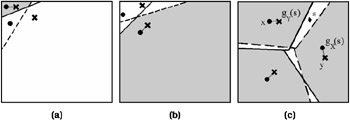Figure 28.2: Three examples of VVS.

As the Basic ViSig method estimates IVS based on uniformly-distributed SV's, the variation in the sizes of Voronoi Cells affects the accuracy of the estimation. One possible method to amend the Basic ViSig method is to generate SV's based on a probability distribution such that the probability of a SV being in a Voronoi Cell is independent of the size of the Cell. Specifically, for two video sequences X and Y, we can define the Probability Density Function (PDF) based on the distribution of Voronoi Cells in [X Y]ε at an arbitrary feature vector u as follows:

 (28.10)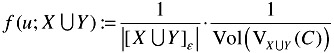where C is the cluster in [X Y]ε with u VXY(C). f(u;X Y) is constant within the Voronoi Cell of each cluster, with the value inversely proportional to the volume of that Cell. Under this PDF, the probability of a random vector u inside the Voronoi Cell VXY(C) for an arbitrary cluster C [X Y]ε is given byf(u; X Y) du = 1/|[X Y]ε|. This probability does not depend on C, and thus, it is equally likely for u to be inside the Voronoi Cell of any cluster in [X Y]ε.

Recall that if we use uniform distribution to generate random SV's, VSSb forms an unbiased estimate of the VVS defined in Equation (28.6). If we use f(u; X Y) to generate SV's instead, VSSb now becomes an estimate of the following general form of VVS:

 (28.11)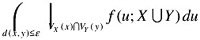Equation (28.11) reduces to Equation (28.6) when f(u; X Y) is replaced by the uniform distribution, i.e., f(u; X Y) = 1. It turns out, as shown by the following proposition, that this general form of VVS is equivalent to the IVS under certain conditions.

##### Proposition 3

Assume we are given two video sequences X and Y. Assume clusters in [X]ε and clusters in [Y]ε either are identical, or share no frames that are within ε from each other. Then, the following relation holds:

 (28.12)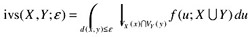The significance of this proposition is that if we can generate SV's with f(u; X Y), it is possible to estimate IVS using VSSb. The condition that all clusters in X and Y are either identical or far away from each other is to avoid the formation of a special region in the feature space called a Voronoi Gap (VG). The concept of VG is expounded in Section 2.5.

In practice, it is impossible to use f(u; X Y) to estimate the IVS between X and Y. This is because f(u; X Y) is specific to the two video sequences being compared, while the Basic ViSig method requires the same set of SV's to be used by all video sequences in the database. A heuristic approach for SV generation is to first select a set Ψ of training video sequences that resemble video sequences in the target database. Denote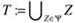. We can then generate SV based on the PDF f(u;T), which ideally resembles the target f(u; X Y) for an arbitrary pair of X and Y in the database.

To generate a random SV s based on f(u;T), we follow a four-step algorithm, called the SV Generation method, as follows:

1. Given a particular value of εSV, identify all the clusters in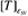using the single-link algorithm.

2. As f(u;T) assigns equal probability to the Voronoi Cell of each cluster in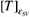, randomly select a cluster C' from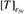so that we can generate the SV s within VT(C').

3. As f(u;T) is constant over VT(C'), we should ideally generate s as a uniformly-distributed random vector over VT(C'). Unless VT(C') can be easily parameterized, the only way to achieve this is to repeatedly generate uniform sample vectors over the entire feature space until a vector is found inside VT(C'). This procedure may take an exceedingly long time if VT(C') is small. To simplify the generation, we select one of the frames in C' at random and output it as the next SV s.

4. Repeat the above process until the required number of SV's has been selected.

In Section 3, we compare performance of this algorithm against uniformly distributed SV generation in retrieving real video sequences.

### 2.5 Voronoi Gap

We show in Proposition 3 that the general form of VVS using an appropriate PDF is identical to the IVS, provided that all clusters between the two sequences are either identical or far away from each other. As feature vectors are not perfect in modeling a human visual system, visually similar clusters may result in feature vectors that are close but not identical to each other. Let us consider the example in Figure 28.2(c) where frames in Similar Clusters between two video sequences are not identical but within ε from each other. Clearly, the IVS is one. Consider the Voronoi Diagrams of the two sequences. Since the boundaries of the two Voronoi Diagrams do not exactly coincide with each other, the Similar Voronoi Region, as indicated by the shaded area, does not occupy the entire feature space. As the general form of VVS defined in Equation (28.11) is the weighted volume of the Similar Voronoi Region, it is strictly less than the IVS. The difference between the two similarities is due to the unshaded region in Figure 28.2(c). The larger the unshaded region is, the larger the difference between the two similarities. If a SV s falls within the unshaded region in Figure 28.2(c), we can make two observations about the corresponding ViSig frames gX(s) and gY(s) from the two sequences X and Y : (1) they are far apart from each other, i.e., d(gX(s), gY(s)) > ε; (2) they both have similar frames in the other video, i.e., there exists x X and y Y such that d(x,gX(s)) ε and d(y,gY(s)) ε. These observations define a unique characteristic of a particular region, which we refer to as Voronoi Gap (VG). Intuitively, any SV in VG between two sequences produces a pair of dissimilar ViSig frames, even though both ViSig frames have a similar match in the other video. More formally, we define VG as follows:

##### Definition 3. Voronoi Gap (VG)

Let X and Y be two video sequences. The VG G(X,Y;ε) of X and Y is defined by all s F that satisfy the following criteria

1. d(gX(s),gY(s)) > ε,

2. there exists x X such that d(x,gY(s)) ε,

3. there exists y Y such that d(y, gX(s)) ε.

In section 2.6, we show that the volume of VG is non-trivial by computing its volume for a particular feature space, namely the Hamming Cube. This implies that it is quite possible to find some of the SV's used in the Basic ViSig method to fall inside the VG. As a result, the performance of the Basic ViSig method in estimating IVS may be adversely affected. In Section 2.7, we introduce the Ranked ViSig method, which mitigates this problem by avoiding SV's that are likely to be inside a VG.

### 2.6 Voronoi Gap in Hamming Cube

The example in Figure 28.2(c) seems to suggest that VG is small if ε is small. An important question is how small ε should be before we can ignore the contribution of the VG. It is obvious that the precise value of the volume of a VG depends on the frame distributions of the two video sequences, and the geometry of the feature space. It is, in general, difficult to compute even a bound on this volume without assuming a particular feature space geometry and frame distributions. In order to get a rough idea of how large VG is, we compute a simple example using a h -dimensional Hamming cube. A Hamming cube is the set containing all the h -bit binary numbers. The distance between two vectors is simply the number of bit-flips to change the binary representation of one vector to the other. Since it is a finite space, the volume function is simply the cardinality of the subset divided by 2h. We choose the Hamming cube because it is easy to analyze, and some commonly used metrics such as l1 and l2 can be embedded inside the Hamming cube with low distortion .

To simplify the calculations, we only consider two-frame video sequences in the h -dimensional Hamming cube H. Let X = {x1,x2} be a video in H. Let the distance between x1 and x2 be a positive integer k. We assume the two frames in X are not similar, i.e., the distance between them is much larger than ε. In particular, we assume that k > 2ε. We want to compute the "gap volume", i.e., the probability of choosing a SV s that is inside the VG formed between X and some video sequence in H. Based on the definition of VG, if a two-frame video sequence Y has a non-empty VG with X, Y must have a frame similar to each frame in X. In other words, the IVS between X and Y must be one. Let Γ be the set of all two-frame sequences whose IVS with X is one. The gap volume is thus the volume of the union of the VG formed between X and each video in Γ. As shown by the following proposition, this gap probability can be calculated using the binomial distribution.

##### Proposition 4

Let X = {x1,x2} be a two-frame video in the Hamming cube H, and Γ be the set of all two-frame sequences whose IVS with X is one. Define A to be the union of the VG formed between X and every video in Γ, i.e.,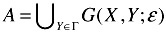Then, if k = d(x1,x2) is an even number larger than 2ε, the volume of A can be computed as follows:

 (28.13)where R is a random variable that follows a binomial distribution with parameters k and 1/2.

We compute Vol(A) numerically by using the right hand side of Equation (28.13). The resulting plot of Vol(A) versus the distance k between the frames in X for ε = 1, 5 ,10 is shown in Figure 28.3(a). Vol(A) decreases as k increases and as ε decreases, but it is hardly insignificant even when k is substantially larger than ε. For example, at k = 500 and ε = 5, Vol(A) 0.34. It is unclear whether the same phenomenon occurs for other feature spaces. Nonetheless, rather than assuming that all VG's are insignificant and using any random SV, intuitively it makes sense to identify those SV's that are inside the VG, and discard them when we estimate the corresponding video similarity.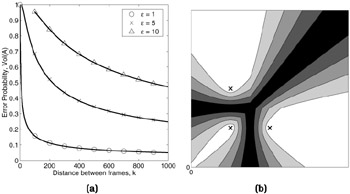Figure 28.3: (a) The error probability for the Hamming cube at different values of ε and distances k between the frames in the video. (b) Values of ranking function Q() for a three-frame video sequence. Lighter colors correspond to larger values.

### 2.7 Ranked Visig Method

Consider again the example in Figure 28.2(c). Assume that we generate m random SV's to compute VSSb. If n out of m SV's are inside the unshaded VG, we can reject these n SV's and use the remaining (m - n) SV's for the computation. The resulting VSSb becomes one which exactly matches the IVS in this example. The only caveat in this approach is that we need an efficient algorithm to determine whether a SV is inside the VG. Direct application of Definition 3 is not very practical, because conditions (2) and (3) in the definition require computing the distances between a ViSig frame of one video and all the frames in the other video. Not only is the time complexity of comparing two ViSig's significantly larger than the VSSb, it defeats the very purpose of using a compact ViSig to represent a video. A more efficient algorithm is thus needed to identify if a SV is inside the VG.

In this section, we propose an algorithm, applied after generating the ViSig, that can identify those SV's which are more likely to be inside the VG. In Figure 28.2(c), we observe that the two sequences have a pair of dissimilar frames that are roughly equidistant from an arbitrary vector s in the VG: x and gX(s) in the "dot" sequence, and y and gY(s) in the "cross" sequence. They are not similar as both d(x,gX(s)) and d(y,gY(s)) are clearly larger than ε. Intuitively, since vectors such as s inside the VG are close to the boundaries between Voronoi Cells in both sequences, it is not surprising to find dissimilar frames such as x and gX(s) that are on either side of the boundaries to be roughly equidistant to s. This "equidistant" condition is refined in the following proposition to upper-bound the difference between distance of s and x, and distance of s and gX(s) by 2ε:

##### Proposition 5

Let X and Y be two video sequences. Assume all clusters in [XY]ε are ε - compact. If a SV s G(X,Y;ε), there exists a frame x X such that

1. x is not similar to gX(s), the ViSig frame in X with respect to s, i.e., d(gx(s),x) > ε.

2. x and gX(s) are roughly equidistant to s. Specifically, d(x,s) - d(gX(s),s) 2ε.

Similarly, we can find a y Y that share the same properties with gY(s).

The significance of Proposition 5 is that it provides a test for determining whether a SV s can ever be inside the VG between a particular video X and any other arbitrary sequence. Specifically, if there does not exist a frame x in X such that x is dissimilar to gX(s) and d(x,s) is within 2ε from d(s,gX(s)), we can guarantee that s will never be inside the VG formed between X and any other sequence. The condition that all Similar Clusters must be ε -compact is to avoid pathological chain-like clusters as discussed in Section 2.1. Such an assumption is not unrealistic for real-life video sequences.

To apply Proposition 5 in practice, we first define a Ranking Function Q( ) for the ViSig frame gX(s),

 (28.14)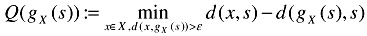An example of Q( ) as a function of a 2-D SV s is shown as a contour plot in Figure 28.3(b). The three crosses represent the frames of a video. Lighter color regions correspond to the area with larger Q( ) values, and thus farther away from the boundaries between Voronoi Cells. By Proposition 5, if Q(gX(s)) > 2ε, s cannot be inside the VG formed between X and any other sequence. In practice, however, this condition might be too restrictive in that it might not allow us to find any SV. Recall that Proposition 5 only provides a sufficient and not a necessary condition for a SV to be inside VG. Thus, even if Q(gx(s)) 2ε, it does not necessarily imply that s will be inside the VG between X and any particular sequence.

Intuitively, in order to minimize the chances of being inside any VG, it makes sense to use a SV s with as large of a Q(gx(s)) value as possible. As a result, rather than using only the ViSig frames with Q(gX(s)) > 2ε, we generate a large number of ViSig frames for each ViSig, and use the few ViSig frames with the largest Q(gX(s)) for similarity measurements. Let m' > m be the number of frames in each ViSig. After we generate the ViSig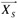by using a set S of m' SV's, we compute and rank Q(gX(s)) for all gX(s) in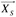. Analogous to VSSb defined in Equation (28.7), we define the Ranked ViSig Similarity (VSSr) between two ViSig'sandbased on their top-ranked ViSig frames:

 (28.15)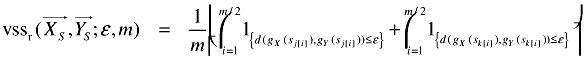j,..., j[m'] and k,,k[m'] 's denote the rankings of the ViSig frames inand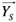respectively, i.e., Q(gX(sj))Q(gX(sj))Q(gX(sj[m])) and Q(gY(Sk))Q(gY(Sk))≥…≥Q(gY(Sk[m']). We call this method of generating ViSig and computing VSSr the Ranked ViSig method. Notice that in the right hand side of Equation (28.15), the first term uses the top-ranked m/2 ViSig frames from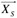to compare with the corresponding ViSig frames in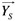, and the second term uses the top-ranked m/2 frames from. Computing VSSr thus requires m metric computations, the same as computing VSSb. This provides an equal footing in complexity to compare the retrieval performances between these two methods in Section 3.

For all x, y in a metric space F , the function d(x, y) is a metric if a) d(x, y) 0 ; b) d(x, y) = 0 =x y ; c) d(x, y) = d(y,x); d) d(x, y) d(x, z) + d(z, y), for all z

If there are multiple x's in X that are equidistant to s, we choose gX(s) to be the one closest to a predefined vector in the feature space such as the origin. If there are still multiple candidates, more predefined vectors can be used until a unique gX(s) is obtained. Such an assignment strategy ensures that gX(s) depends only on X and s but not some arbitrary random choices. This is important to the ViSig method which uses gX(s) as part of a summary of X with respect to a randomly selected s. Since gX(s) depends only on X and s, sequences identical to X produce the same summary frame with respect to s.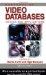Handbook of Video Databases: Design and Applications (Internet and Communications)
ISBN: 084937006X
EAN: 2147483647
Year: 2003
Pages: 393

flylib.com © 2008-2017.
If you may any questions please contact us: flylib@qtcs.net